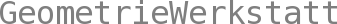Konforme Feldtheorie
SS 2011  •  info

Prof. Dr. Christoph Bohle
email
Dr. Sebastian Heller

Vorlesung
Ab der 2. Vorlesungswoche
 Fr 12-14

Übung
 Mi 12-14 S8
Beginn der Übung: 3. Vorlesungswoche
Übungsblätter

One of the major problems of contemporary mathematics is to develop a better understanding of quantum field theory, the theory on which large parts of fundamental physics are based. Despite its success as a physical theory, much of quantum field theory in its present form is lacking a rigorous mathematical foundation. This situation is highly dissatisfactory, considering that in the last decades quantum field theory turned into a preeminent source of mathematical inspiration.

Conformal field theory is a part of quantum field theory which allows a mathematically rigorous treatment. Although is encompasses only a small portion of quantum field theory (that of 2D quantum field theories with local conformal symmetry), it is a good starting point for mathematicians to get acquainted with quantum field theory ideas. This is not only due to its mathematical accessibility, but also because of its connection to several important mathematical developments.

We will essentially approach conformal field theory as a mathematical theory. The main part of the lecture will be an introduction to vertex algebras, the algebraic structure underlying conformal field theory. In the last part of the lecture we plan to discuss some mathematical applications related to integrable systems and the theory of vector bundles on Riemann surfaces. We shamefully ignore all physical applications (although they are plenty, most prominently in string theory and statistical mechanics, where conformal field theory describes critical phenomena).

Prerequisites: A basic knowledge of Lie algebras and Riemann surfaces will be helpful. We do not assume any knowledge of physics, although a deeper understanding surely needs some.